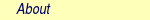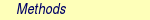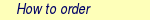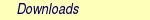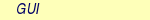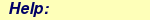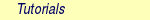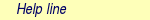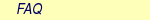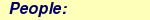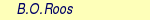MOLCAS manual:Next: 10.3 Computing a reaction path. Up: 10. Examples Previous: 10.1 Computing high symmetry molecules.

Subsections

# 10.2 Geometry optimizations and Hessians.

To optimize a molecular geometry is probably one of the most frequent interests of a quantum chemist . In the present section we examine some examples of obtaining stationary points on the energy surfaces. We will focus in this section in searching of minimal energy points, postponing the discussion on transition states to section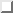. This type of calculations require the computation of molecular gradients, whether using analytical or numerical derivatives. We will also examine how to obtain the full geometrical Hessian for a molecular state, what will provide us with vibrational frequencies within the harmonic approximation and thermodynamic properties by the use of the proper partition functions.

The program ALASKA computes analytical gradients for optimized wave functions. In 8.1 the SCF, DFT, and CASSCF/RASSCF levels of calculation are available. The program ALASKA also computes numerical gradients from CASPT2 and MS-CASPT2 energies. Provided with the first order derivative matrix with respect to the nuclei and an approximate guess of the Hessian matrix, the program SLAPAF is then used to optimize molecular structures. From MOLCAS-5 it is not necessary to explicitly define the set of internal coordinates of the molecule in the SLAPAF input. Instead a redundant coordinates approach is used. If the definition is absent the program builds its own set of parameters based on curvature-weighted non-redundant internal coordinates and displacements . As they depend on the symmetry of the system it might be somewhat difficult in some systems to define them. It is, therefore, strongly recommended to let the program define its own set of non-redundant internal coordinates. In certain situations such as bond dissociations the previous coordinates may not be appropriate and the code directs the user to use instead Cartesian coordinates, for instance.

## 10.2.1 Ground state optimizations and vibrational analysis

As an example we are going to work with the 1,3-cyclopentadiene molecule. This is a five-carbon system forming a ring which has two conjugated double bonds. Each carbon has one attached hydrogen atom except one which has two. We will use the CASSCF method and take advantage of the symmetry properties of the molecule to compute ground and excited states. To ensure the convergence of the results we will also perform Hessian calculations to compute the force fields at the optimized geometries.

In this section we will combine two types of procedures to perform calculations in MOLCAS. The user may then choose the most convenient for her/his taste. We can use an general script and perform an input-oriented calculation, when all the information relative to the calculation, including links for the files and control of iterations, are inserted in the input file. The other procedure is the classical script-oriented system used in previous examples and typically previous versions of MOLCAS. Let's start by making an input-oriented optimization. A script is still needed to perform the basic definitions, although they can be mostly done within the input file. A suggested form for this general script could be:

#!/bin/sh
export MOLCAS=/home/molcas/molcashome
export MOLCAS_MEM=64
export HomeDir=/home/somebody/somewhere
export WorkDir=$HomeDir/$Project
[ ! -d $WorkDir ] && mkdir$WorkDir
molcas $HomeDir/$Project.input >$HomeDir/$Project.out 2>$HomeDir/$Project.err
exit

We begin by defining the input for the initial calculation. In simple cases the optimization procedure is very efficient. We are going, however, to design a more complete procedure that may help in more complex situations. It is sometimes useful to start the optimization in a small size basis set and use the obtained approximate Hessian to continue the calculation with larger basis sets. Therefore, we will begin by using the minimal STO-3G basis set to optimize the ground state of 1,3-cyclopentadiene within C2v symmetry.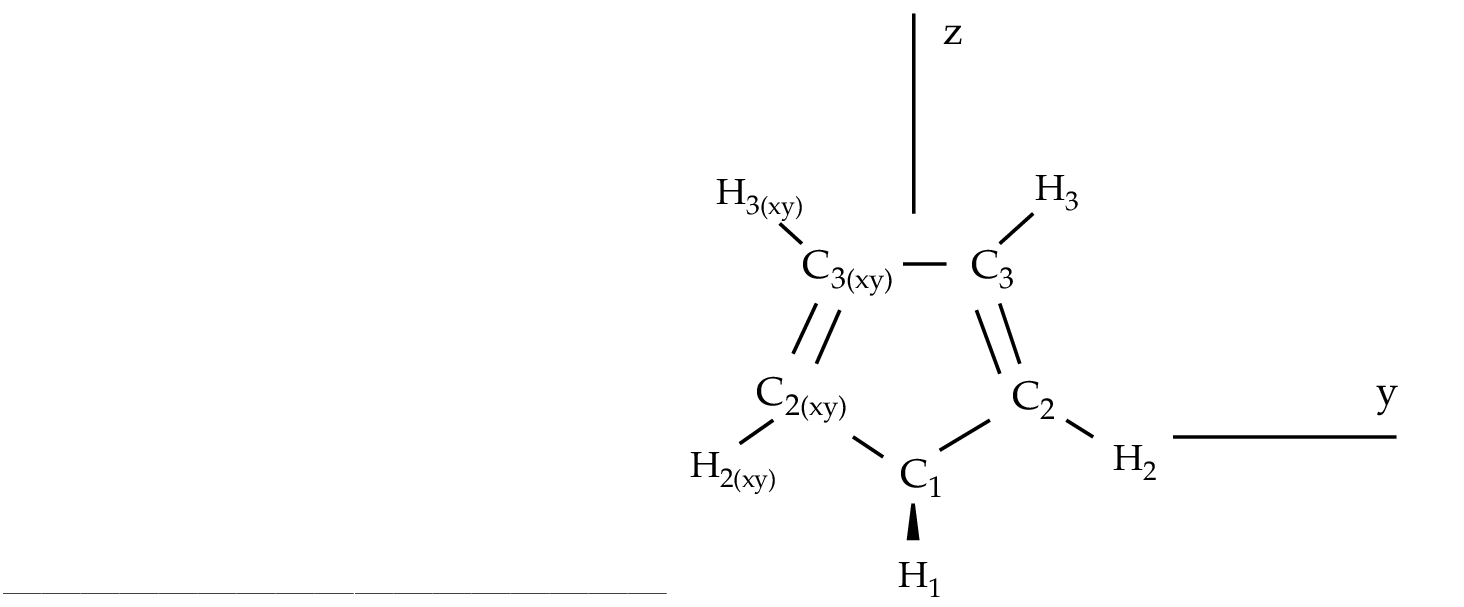>>>  EXPORT  MOLCAS_MAXITER=50
Symmetry=  X  XY
Basis  set
C.STO-3G....
C1  0.000000  0.000000  0.000000  Bohr
C2  0.000000  2.222644  1.774314  Bohr
C3  0.000000  1.384460  4.167793  Bohr
End  of  basis
Basis  set
H.STO-3G....
H1  1.662033  0.000000  -1.245623  Bohr
H2  0.000000  4.167844  1.149778  Bohr
H3  0.000000  2.548637  5.849078  Bohr
End  of  basis

>>>  Do  while  <<<

&SEWARD

>>>  IF  (  ITER  =  1  )
&SCF
OCCUPIED=9  1  6  2
ITERATIONS=40
>>>  END  IF
&RASSCF
SYMMETRY=1;  SPIN=1
NACTEL=  6  0  0
INACTIVE=  9  0  6  0
RAS2=  0  2  0  3  <---  All  pi  valence  orbitals  active
ITER=  50,25;  CIMX=  25

&SLAPAF;  Iterations=80;  Thrs=0.5D-06  1.0D-03
>>>  EndDo  <<<
>>>  COPY  $Project.RunFile$CurrDir/$Project.ForceConstant.STO-3G A copy of the RUNFILE has been made at the end of the input stream. This saves the file for use as (a) starting geometry and (b) a guess of the Hessian matrix in the following calculation. The link can be also done in the shell script. The generators used to define the C2v symmetry are X and XY, plane yz and axis z. They differ from those used in other examples as in section. The only consequence is that the order of the symmetries in SEWARD differs. In the present case the order is: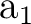,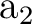,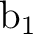, and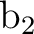, and consequently the classification by symmetries of the orbitals in the SCF and RASSCF inputs will differ. It is therefore recommended to initially use the option TEST in the GATEWAY input to check the symmetry option. This option, however, will stop the calculation after the GATEWAY input head is printed. The calculation converges in four steps. We change now the input. We can choose between replacing by hand the geometry of the SEWARD input or use the same$WorkDir directory and let the program to take the last geometry stored into the RUNFILE file. In any case the new input can be:

>>COPY  $CurrDir/OPT.hessian.ForceConstant.STO-3G$Project.RunOld

Symmetry=X  XY
Basis  set
C.ANO-L...4s3p1d.
C1  .0000000000  .0000000000  -2.3726116671
C2  .0000000000  2.2447443782  -.5623842095
C3  .0000000000  1.4008186026  1.8537195887
End  of  basis
Basis  set
H.ANO-L...2s.
H1  1.6523486260  .0000000000  -3.6022531906
H2  .0000000000  4.1872267035  -1.1903003793
H3  .0000000000  2.5490335048  3.5419847446
End  of  basis

>>>  Do  while  <<<

&SEWARD

>>>  IF  (  ITER  =  1  )  <<<<
&SCF
OCCUPIED=  9  1  6  2
ITERATIONS=  40

>>>  ENDIF  <<<

SYMMETRY=1;  SPIN=1;  NACTEL=6  0  0
INACTIVE=  9  0  6  0
RAS2  =  0  2  0  3
ITER=50,25;  CIMX=  25

&SLAPAF;  Iterations=80;  Thrs=0.5D-06  1.0D-03
OldForce  Constant  Matrix
>>>  EndDo  <<<

The RUNOLD file will be used by SEWARD to pick up the molecular structure on the initial iteration and by SLAPAF as initial Hessian to carry out the relaxation. This use of the RUNFILE can be done between any different calculations provided they work in the same symmetry.

In the new basis set, the resulting optimized geometry at the CASSCF level in C2v symmetry is:

********************************************
* Values of internal coordinates *
********************************************
C2C1 2.851490 Bohr
C3C2 2.545737 Bohr
C3C3 2.790329 Bohr
H1C1 2.064352 Bohr
H2C2 2.031679 Bohr
H3C3 2.032530 Bohr
C1C2C3 109.71 Degrees
C1C2H2 123.72 Degrees
C2C3H3 126.36 Degrees
H1C1H1 107.05 Degrees

Once we have the optimized geometry we can obtain the force field, to compute the force constant matrix and obtain an analysis of the harmonic frequency. This is done by computing the analytical Hessian at the optimized geometry. Notice that this is a single-shot calculation using the MCKINLEY, which will automatically start the MCLR module in case of a frequency calculation.

Symmetry=  X  XY
Basis  set
C.ANO-L...4s3p1d.
C1  0.0000000000  0.0000000000  -2.3483061484
C2  0.0000000000  2.2245383122  -0.5643712787
C3  0.0000000000  1.3951643642  1.8424767578
End  of  basis
Basis  set
H.ANO-L...2s.
H1  1.6599988023  0.0000000000  -3.5754797471
H2  0.0000000000  4.1615845660  -1.1772096132
H3  0.0000000000  2.5501642966  3.5149458446
End  of  basis

&SEWARD
OCCUPIED=  9  1  6  2
ITERATIONS=  40
SYMMETRY=1;  SPIN=1;  NACTEL=  6  0  0
INACTIVE=  9  0  6  0
RAS2  =  0  2  0  3
ITER=  50,25;  CIMX=25

&MCKINLEY

Cyclopentadiene has 11 atoms, that mean 3N = 33 Cartesian degrees of freedom. Therefore the MCLR output will contain 33 frequencies. From those, we are just interested in the 3N-6 = 27 final degrees of freedom that correspond to the normal modes of the system. We will discard from the output the three translational (Ti) and three rotational (Ri) coordinates. The table of characters gives us the classification of these six coordinates: a1 (Tz), a2 (Rz), b2 (Tx,Ry), b1 (Ty,Rx). This information is found in the Seward output:

Character Table for C2v

E s(yz) C2(z) s(xz)
a1 1 1 1 1 z
a2 1 -1 1 -1 xy, Rz, I
b2 1 1 -1 -1 y, yz, Rx
b1 1 -1 -1 1 x, xz, Ry

It is simply to distinguish these frequencies because they must be zero, although and because of numerical inaccuracies they will be simply close to zero. Note that the associated intensities are nonsense. In the present calculation the harmonic frequencies, the infrared intensities, and the corresponding normal modes printed below in Cartesian coordinates are the following:

Symmetry a1
==============

1 2 3 4 5 6

Freq. 0.04 847.85 966.08 1044.69 1187.61 1492.42

Intensity: 0.646E-08 0.125E-02 0.532E+01 0.416E+00 0.639E-01 0.393E+01

C1 z 0.30151 0.35189 -0.21166 -0.11594 0.06874 0.03291
C2 y 0.00000 0.31310 0.14169 0.12527 -0.01998 -0.08028
C2 z 0.30151 -0.02858 0.06838 -0.00260 0.02502 -0.06133
C3 y -0.00000 0.04392 -0.07031 0.23891 -0.02473 0.16107
C3 z 0.30151 -0.15907 0.00312 0.08851 -0.07733 -0.03146
H1 x 0.00000 -0.02843 -0.00113 -0.01161 0.00294 0.04942
H1 z 0.30151 0.31164 -0.21378 -0.13696 0.08233 0.11717
H2 y 0.00000 0.24416 0.27642 0.12400 0.11727 0.07948
H2 z 0.30151 -0.25054 0.46616 -0.05986 0.47744 0.46022
H3 y -0.00000 -0.29253 -0.28984 0.59698 0.34878 -0.34364
H3 z 0.30151 0.07820 0.15644 -0.13576 -0.34625 0.33157

7 8 9 10 11

Freq. 1579.76 1633.36 3140.69 3315.46 3341.28

Intensity: 0.474E+01 0.432E+00 0.255E+02 0.143E+02 0.572E+01
...

Symmetry a2
==============

1 2 3 4 5

Freq. i9.26 492.62 663.74 872.47 1235.06

...

Symmetry b2
==============

1 2 3 4 5 6

Freq. i10.61 0.04 858.72 1020.51 1173.33 1386.20

Intensity: 0.249E-01 0.215E-07 0.259E+01 0.743E+01 0.629E-01 0.162E+00

...
7 8 9 10

Freq. 1424.11 1699.07 3305.26 3334.09

Intensity: 0.966E+00 0.426E+00 0.150E+00 0.302E+02

...

Symmetry b1
==============

1 2 3 4 5 6

Freq. i11.31 0.11 349.15 662.98 881.19 980.54

Intensity: 0.459E-01 0.202E-06 0.505E+01 0.896E+02 0.302E+00 0.169E+02

...

7

Freq. 3159.81

Intensity: 0.149E+02
...

Apart from the six mentioned translational and rotational coordinates There are no imaginary frequencies and therefore the geometry corresponds to a stationary point within the C2v symmetry. The frequencies are expressed in reciprocal centimeters.

After the vibrational analysis the zero-point energy correction and the thermal corrections to the total energy, internal, entropy, and Gibbs free energy. The analysis uses the standard expressions for an ideal gas in the canonical ensemble which can be found in any standard statistical mechanics book. The analysis is performed at different temperatures, for instance:

*****************************************************
Temperature  =  273.00  Kelvin,  Pressure  =  1.00  atm
-----------------------------------------------------
Molecular  Partition  Function  and  Molar  Entropy:
q/V  (M**-3)  S(kcal/mol*K)
Electronic  0.100000D+01  0.000
Translational  0.143889D+29  38.044
Rotational  0.441593D+05  24.235
Vibrational  0.111128D-47  3.002
TOTAL  0.706112D-15  65.281

Thermal  contributions  to  INTERNAL  ENERGY:
Electronic  0.000  kcal/mol  0.000000  au.
Translational  0.814  kcal/mol  0.001297  au.
Rotational  0.814  kcal/mol  0.001297  au.
Vibrational  60.723  kcal/mol  0.096768  au.
TOTAL  62.350  kcal/mol  0.099361  au.

Thermal  contributions  to
ENTHALPY  62.893  kcal/mol  0.100226  au.
GIBBS  FREE  ENERGY  45.071  kcal/mol  0.071825  au.

Sum  of  energy  and  thermal  contributions
INTERNAL  ENERGY  -192.786695  au.
ENTHALPY  -192.785831  au.
GIBBS  FREE  ENERGY  -192.814232  au.

Next, polarizabilities (see below) and isotope shifted frequencies are also displayed in the output.

************************************
*  *
*  Polarizabilities  *
*  *
************************************

34.76247619
-0.00000000  51.86439359
-0.00000000  -0.00000000  57.75391824

For a graphical representation of the harmonic frequencies one can also use the $Project.freq.molden file as an input to the MOLDEN program. ## 10.2.2 Excited state optimizations The calculation of excited states using the ALASKA and SLAPAF codes has no special characteristic. The wave function is defined by the SCF or RASSCF programs. Therefore if we want to optimize an excited state the RASSCF input has to be defined accordingly. It is not, however, an easy task, normally because the excited states have lower symmetry than the ground state and one has to work in low order symmetries if the full optimization is pursued. Take the example of the thiophene molecule (see fig.in next section). The ground state has C2v symmetry: 1 1A1. The two lowest valence excited states are 21A1 and 11B2. If we optimize the geometries within the C2v symmetry the calculations converge easily for the three states. They are the first, second, and first roots of their symmetry, respectively. But if we want to make a full optimization in C1, or even a restricted one in Cs, all three states belong to the same symmetry representation. The higher the root more difficult is to converge it. A geometry optimization requires single-root optimized CASSCF wave-functions, but, unlike in previous MOLCAS versions, we can now carry out State-Average (SA) CASSCF calculations between different roots. The wave functions we have with this procedure are based on an averaged density matrix, and a further orbital relaxation is required. The MCLR program can perform such a task by means of a perturbational approach. Therefore, if we choose to carry out a SA-CASSCF calculations in the optimization procedure, the Alaska module will automatically start up the MCLR module. We are going to optimize the three states of thiophene in C2v symmetry. The inputs are: &GATEWAY; Title=Thiophene molecule Symmetry= X XY Basis set S.ANO-S...4s3p2d. S1 .0000000000 .0000000000 -2.1793919255 End of basis Basis set C.ANO-S...3s2p1d. C1 .0000000000 2.3420838459 .1014908659 C2 .0000000000 1.3629012233 2.4874875281 End of basis Basis set H.ANO-S...2s. H1 .0000000000 4.3076765963 -.4350463731 H2 .0000000000 2.5065969281 4.1778544652 End of basis >>> Do while <<< &SEWARD >>> IF ( ITER = 1 ) <<< &SCF; TITLE=Thiophene molecule OCCUPIED= 11 1 7 3 ITERATIONS= 40 >>> ENDIF <<< &RASSCF; TITLE=Thiophene molecule 1 1A1 SYMMETRY=1; SPIN=1; NACTEL= 6 0 0 INACTIVE= 11 0 7 1 RAS2 = 0 2 0 3 ITER= 50,25 &ALASKA &SLAPAF End of Input >>> ENDDO <<< for the ground state. For the two excited states we will replace the RASSCF inputs with &RASSCF; TITLE=Thiophene molecule 2 1A1 SYMMETRY=1; SPIN=1; NACTEL= 6 0 0 INACTIVE= 11 0 7 1 RAS2 = 0 2 0 3 ITER= 50,25 CIROOT= 2 2; 1 2; 1 1 LEVSHFT=1.0 RLXRoot= 2 for the 21A1 state. Notice that we are doing a SA-CASSCF calculation including two roots, therefore we must use the keyword RLXROOT within the RASSCF input to specify for which state we want the root. We have also &RASSCF; TITLE=Thiophene molecule 1 1B2 SYMMETRY=2; SPIN=1; NACTEL= 6 0 0 INACTIVE= 11 0 7 1 RAS2 = 0 2 0 3 ITER= 50,25 LEVSHFT=1.0 for the 11B2 state. To help the program to converge we can include one or more initial RASSCF inputs in the input file. The following is an example for the calculation of the of the 31A' state of thiophene (Cs symmetry) with a previous calculation of the ground state to have better starting orbitals. &GATEWAY; Title= Thiophene molecule Symmetry=X Basis set S.ANO-S...4s3p2d. S1 .0000000000 .0000000000 -2.1174458547 End of basis Basis set C.ANO-S...3s2p1d. C1 .0000000000 2.4102089951 .1119410701 C1b .0000000000 -2.4102089951 .1119410701 C2 .0000000000 1.3751924147 2.7088559532 C2b .0000000000 -1.3751924147 2.7088559532 End of basis Basis set H.ANO-S...2s. H1 .0000000000 4.3643321746 -.4429940876 H1b .0000000000 -4.3643321746 -.4429940876 H2 .0000000000 2.5331491787 4.3818833166 H2b .0000000000 -2.5331491787 4.3818833166 End of basis >>> Do while <<< &SEWARD >>> IF ( ITER = 1 ) <<< &SCF; TITLE= Thiophene molecule OCCUPIED= 18 4 ITERATIONS = 40 &RASSCF; TITLE= Thiophene molecule 1A' SYMMETRY=1; SPIN=1; NACTEL= 6 0 0 INACTIVE= 18 1 RAS2 = 0 5 ITER= 50,25 >>> ENDIF <<< &RASSCF; TITLE= Thiophene molecule 3 1A' SYMMETRY=1; SPIN=1; NACTEL= 6 0 0 INACTIVE= 18 1 RAS2 = 0 5 ITER= 50,25 CIROOT=3 3 1 RLXRoot= 3 &ALASKA &SLAPAF &END >>> ENDDO <<< It should be remembered that geometry optimizations for excited states are difficult. Not only can it be difficult to converge the corresponding RASSCF calculation, but we must also be sure that the order of the states does not change during the optimization of the geometry. This is not uncommon and the optimization must be followed by the user. ## 10.2.3 Restrictions in symmetry or geometry. ### 10.2.3.1 Optimizing with geometrical constraints. A common situation in geometry optimizations is to have one or several coordinates fixed or constrained and vary the remaining coordinates. As an example we will take the biphenyl molecule, two benzene moieties bridged by a single bond. The ground state of the molecule is not planar. One benzene group is twisted by 44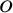degrees with respect to the other . We can use this example to perform two types of restricted optimizations. The simplest way to introduce constraints is to give a coordinate a fixed value and let the other coordinates to be optimized. For instance, let's fix the dihedral angle between both benzenes to be fixed to 44degrees. Within this restriction, the remaining coordinates will be fully optimized. The Constraints keyword in the program GATEWAY will take care of the restriction (note this keyword could also be placed in the program SLAPAF). The input could be: &GATEWAY; Title= Biphenyl twisted D2 Symmetry= XY XZ Basis set C.ANO-S...3s2p1d. C1 1.4097582886 .0000000000 .0000000000 C2 2.7703009377 2.1131321616 .8552434921 C3 5.4130377085 2.1172148045 .8532344474 C4 6.7468359904 .0000000000 .0000000000 End of basis Basis set H.ANO-S...2s. H2 1.7692261798 3.7578798540 1.5134152112 H3 6.4188773347 3.7589592975 1.5142479153 H4 8.7821560635 .0000000000 .0000000000 End of basis Constraints d1 = Dihedral C2 C1 C1(XY) C2(XY) Values d1 = -44.4 degrees End of Constraints >>> Do while <<< &SEWARD >>> IF ( ITER = 1 ) <<< &SCF; TITLE= Biphenyl twisted D2 OCCUPIED= 12 9 9 11 ITERATIONS= 50 >>> ENDIF <<< &RASSCF; TITLE= Biphenyl twisted D2 SYMMETRY=1; SPIN=1; NACTEL= 12 0 0 INACTIVE= 11 7 7 10 RAS2 = 2 4 4 2 &ALASKA &SLAPAF; Iterations=30; MaxStep=1.0 >>> ENDDO <<< One important consideration about the constraint. You do not need to start at a geometry having the exact value for the coordinate you have selected (44.4 degrees for the dihedral angle here). The optimization will lead you to the right solution. On the other hand, if you start exactly with the dihedral being 44.4 deg the code does not necessarily will freeze this value in the first iterations, but will converge to it at the end. Therefore, it may happen that the value for the dihedral differs from the selected value in the initial iterations. You can follow the optimization steps in the$WorkDir directory using the MOLDEN files generated automatically by MOLCAS.

Now we will perform the opposite optimization: we want to optimize the dihedral angle relating both benzene units but keep all the other coordinates fixed. We could well use the same procedure as before adding constraints for all the remaining coordinates different from the interesting dihedral angle, but to build the input would be tedious. Therefore, instead of keyword Constraints we will make use of the keywords Vary and Fix.

The input file should be:

&GATEWAY;  Title=  Biphenyl  twisted  D2
Symmetry=XY  XZ
Basis  set
C.ANO-S...3s2p1d.
C1  1.4097582886  .0000000000  .0000000000
C2  2.7703009377  2.1131321616  .8552434921
C3  5.4130377085  2.1172148045  .8532344474
C4  6.7468359904  .0000000000  .0000000000
End  of  basis
Basis  set
H.ANO-S...2s.
H2  1.7692261798  3.7578798540  1.5134152112
H3  6.4188773347  3.7589592975  1.5142479153
H4  8.7821560635  .0000000000  .0000000000
End  of  basis

>>>  Do  while  <<<
&SEWARD
>>>  IF  (  ITER  =  1  )  <<<
&SCF;  TITLE=  Biphenyl  twisted  D2
OCCUPIED=  12  9  9  11
ITERATIONS=  50
>>>  ENDIF  <<<

&RASSCF;  TITLE=  Biphenyl  twisted  D2
SYMMETRY=1;  SPIN=1;  NACTEL=12  0  0
INACTIVE=  11  7  7  10
RAS2  =  2  4  4  2

&SLAPAF
Internal  coordinates
b1  =  Bond  C1  C1(XY)
b2  =  Bond  C1  C2
b3  =  Bond  C2  C3
b4  =  Bond  C3  C4
h1  =  Bond  C2  H2
h2  =  Bond  C3  H3
h3  =  Bond  C4  H4
a1  =  Angle  C2  C1  C1(XY)
a2  =  Angle  C1  C2  C3
a3  =  Angle  C1  C2  H2
a4  =  Angle  C2  C3  H3
phi  =  Dihedral  C2  C1  C1(XY)  C2(XY)
d1  =  Dihedral  H2  C2  C1  C1(XY)
d2  =  OutOfP  C3  C1(XY)  C1  C2
d3  =  Dihedral  H3  C3  C2  H2
Vary;  phi
Fix;  b1;  b2;  b3;  b4;  h1;  h2;  h3;  a1;  a2;  a3;  a4;  d1;  d2;  d3
End  of  Internal
Iterations=  30
>>>  ENDDO  <<<

To be able to optimize the molecule in that way a D2 symmetry has to be used. In the definition of the internal coordinates we can use an out-of-plane coordinate: C2 C2(xy) C1(xy) C1 or a dihedral angle C2 C1 C1(xy) C2(xy). In this case there is no major problem but in general one has to avoid as much as possible to define dihedral angles close to 180( trans conformation ). The SLAPAF program will warn about this problem if necessary. In the present example, angle 'phi' is the angle to vary while the remaining coordinates are frozen. All this is only a problem in the user-defined internal approach, not in the non-redundant internal approach used by default in the program. In case we do not have the coordinates from a previous calculation we can always run a simple calculation with one iteration in the SLAPAF program.

It is not unusual to have problems in the relaxation step when one defines internal coordinates. Once the program has found that the definition is consistent with the molecule and the symmetry, it can happen that the selected coordinates are not the best choice to carry out the optimization, that the variation of some of the coordinates is too large or maybe some of the angles are close to their limiting values (180for Dihedral angles and90for Out of Plane angles). The SLAPAF program will inform about these problems. Most of the situations are solved by re-defining the coordinates, changing the basis set or the geometry if possible, or even freezing some of the coordinates. One easy solution is to froze this particular coordinate and optimize, at least partially, the other as an initial step to a full optimization. It can be recommended to change the definition of the coordinates from internal to Cartesian.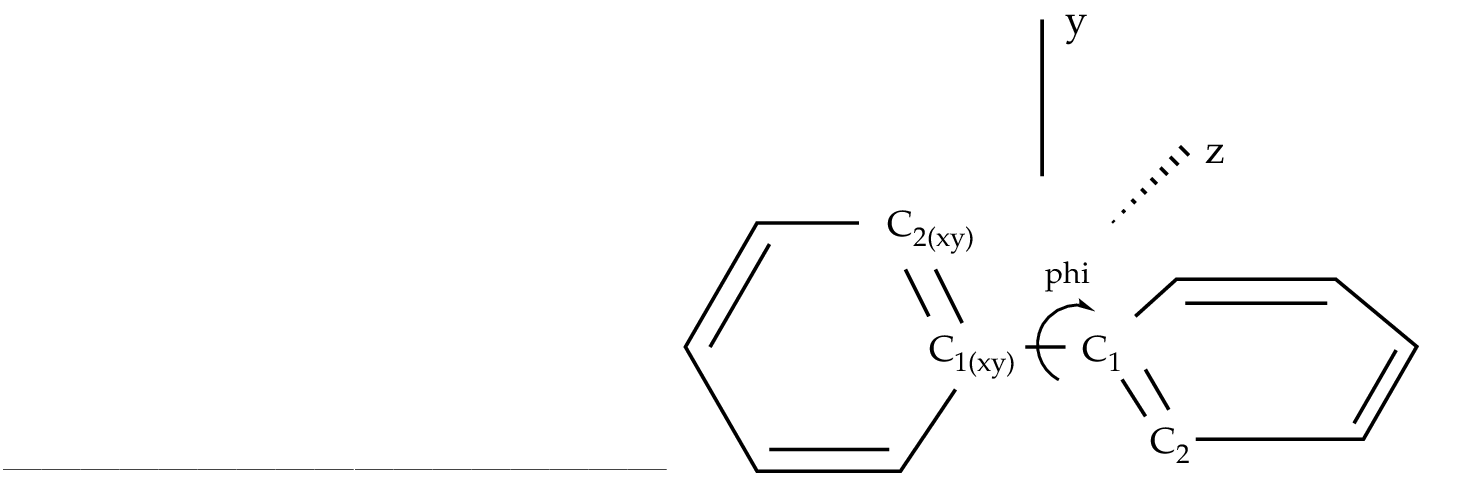### 10.2.3.2 Optimizing with symmetry restrictions.

Presently, MOLCAS is prepared to work in the point groups C1, Ci, Cs, C2, D2, C2h, C2v, and D2h. To have the wave functions or geometries in other symmetries we have to restrict orbital rotations or geometry relaxations specifically. We have shown how to in the RASSCF program by using the SUPSym option. In a geometry optimization we may also want to restrict the geometry of the molecule to other symmetries. For instance, to optimize the benzene molecule which belongs to the D6h point group we have to generate the integrals and wave function in D2h symmetry, the highest group available, and then make the appropriate combinations of the coordinates chosen for the relaxation in the SLAPAF program, as is shown in the manual.

As an example we will take the ammonia molecule, NH3. There is a planar transition state along the isomerization barrier between two pyramidal structures. We want to optimize the planar structure restricted to the D3h point group. However, the electronic wave function will be computed in Cs symmetry (C2v is also possible) and will not be restricted, although it is possible to do that in the RASSCF program.

The input for such a geometry optimization is:

&GATEWAY;  Title=  NH3,  planar
Symmetry=  Z
Basis  Set
N.ANO-L...4s3p2d.
N  .0000000000  .0000000000  .0000000000
End  of  Basis
Basis  set
H.ANO-L...3s2p.
H1  1.9520879910  .0000000000  .0000000000
H2  -.9760439955  1.6905577906  .0000000000
H3  -.9760439955  -1.6905577906  .0000000000
End  of  Basis

>>>  Do  while  <<<
&SEWARD
>>>  IF  (  ITER  =  1  )  <<<
&SCF;  Title=  NH3,  planar
Occupied=  4  1
Iterations=  40
>>>  ENDIF  <<<

&RASSCF;  Title=  NH3,  planar
Symmetry=1;  Spin=1;  Nactel=8  0  0
INACTIVE=1  0
RAS2  =6  2

&SLAPAF
Internal  coordinates
b1  =  Bond  N  H1
b2  =  Bond  N  H2
b3  =  Bond  N  H3
a1  =  Angle  H1  N  H2
a2  =  Angle  H1  N  H3
Vary
r1  =  1.0  b1  +  1.0  b2  +  1.0  b3
Fix
r2  =  1.0  b1  -  1.0  b2
r3  =  1.0  b1  -  1.0  b3
a1  =  1.0  a1
a2  =  1.0  a2
End  of  internal
>>>  ENDDO  <<<

All four atoms are in the same plane. Working in Cs, planar ammonia has five degrees of freedom. Therefore we must define five independent internal coordinates, in this case the three N-H bonds and two of the three angles H-N-H. The other is already defined knowing the two other angles. Now we must define the varying coordinates. The bond lengths will be optimized, but all three N-H distances must be equal. First we define (see definition in the previous input) coordinate r1 equal to the sum of all three bonds; then, we define coordinates r2 and r3 and keep them fixed. r2 will ensure that bond1 is equal to bond2 and r3 will assure that bond3 is equal to bond1. r2 and r3 will have a zero value. In this way all three bonds will have the same length. As we want the system constrained into the D3h point group, the three angles must be equal with a value of 120 degrees. This is their initial value, therefore we simply keep coordinates ang1 and ang2 fixed. The result is a D3h structure:

*******************************************
* InterNuclear Distances / Angstrom *
*******************************************

1 N 2 H1 3 H2 4 H3
1 N 0.000000
2 H1 1.003163 0.000000
3 H2 1.003163 1.737529 0.000000
4 H3 1.003163 1.737529 1.737529 0.000000

**************************************
* Valence Bond Angles / Degree *
**************************************
Atom centers Phi
2 H1 1 N 3 H2 120.00
2 H1 1 N 4 H3 120.00
3 H2 1 N 4 H3 120.00

In a simple case like this an optimization without restrictions would also end up in the same symmetry as the initial input.

## 10.2.4 Optimizing with Z-Matrix.

An alternative way to optimize a structure with geometrical and/or symmetrical constraints is to combine the Z-Matrix definition of the molecular structure used for the program SEWARD with a coherent definition for the Internal Coordinated used in the optimization by program SLAPAF.

Here is an examples of optimization of the methyl carbanion. Note that the wavefunction is calculated within the Cs symmetry but the geometry is optimized within the C3v symmetry through the ZMAT and the Internal Coordinates definitions. Note that XBAS precedes ZMAT.

&Gateway
Symmetry=Y
XBAS=Aug-cc-pVDZ
ZMAT
C1
X2 1 1.00
H3 1 1.09 2 105.
H4 1 1.09 2 105. 3 120.

>>> export MOLCAS_MAXITER=500
>>> Do While <<<

&SEWARD
&SCF; Charge= -1

&SLAPAF
Internal Coordinates
CX2 = Bond C1 X2
CH3 = Bond C1 H3
CH4 = Bond C1 H4
XCH3 = Angle X2 C1 H3
XCH4 = Angle X2 C1 H4
DH4 = Dihedral H3 X2 C1 H4
Vary
SumCH34 = 1. CH3 +2. CH4
SumXCH34 = 1. XCH3 +2. XCH4
Fix
rCX2 = 1.0 CX2
DifCH34 = 2. CH3 -1. CH4
DifXCH34 = 2. XCH3 -1. XCH4
dDH4 = 1.0 DH4
End of Internal
PRFC
Iterations= 10
>>> EndDo <<<

Note that the dummy atom X2 is used to define the Z axis and the planar angles for the hydrogen atoms. The linear combinations of bond distances and planar angles in the expression in the Vary and Fix sections are used to impose the C3v symmetry.

Another examples where the wavefunction and the geometry can be calculated within different symmetry groups is benzene. In this case, the former uses D2h symmetry and the latter D6h symmetry. Two special atoms are used: the dummy X1 atom defines the center of the molecule while the ghost Z2 atom is used to define the C6 rotational axis (and the Z axis).

&GATEWAY
Symmetry=X Y Z
XBAS
H.ANO-S...2s.
C.ANO-S...3s2p.
End of basis
ZMAT
X1
Z2 1 1.00
C3 1 1.3915 2 90.
C4 1 1.3915 2 90. 3 60.
H5 1 2.4715 2 90. 3 0.
H6 1 2.4715 2 90. 3 60.

>>> export MOLCAS_MAXITER=500
>>> Do While <<<

&SLAPAF
Internal Coordinates
XC3 = Bond X1 C3
XC4 = Bond X1 C4
XH5 = Bond X1 H5
XH6 = Bond X1 H6
CXC = Angle C3 X1 C4
HXH = Angle H5 X1 H6
Vary
SumC = 1.0 XC3 + 2.0 XC4
SumH = 1.0 XH5 + 2.0 XH6
Fix
DifC = 2.0 XC3 - 1.0 XC4
DifH = 2.0 XH5 - 1.0 XH6
aCXC = 1.0 CXC
aHXH = 1.0 HXH
End of Internal
PRFC

>>> EndDo <<<

Note that the ghost atom Z2 is used to define the geometry within the Z-Matrix but it does not appear in the Internal Coordinates section. On the other hand, the dummy atom X1 represents the center of the molecule and it is used in the Internal Coordinates section.

## 10.2.5 CASPT2 optimizations

For systems showing a clear multiconfigurational nature, the CASSCF treatment on top of the HF results is of crucial importance in order to recover the large non dynamical correlation effects. On the other hand, ground-state geometry optimizations of closed shell systems are not exempt from non dynamical correlation effects. In general, molecules containing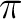-electrons suffer from significant effects of non dynamical correlation, even more in presence of conjugated groups. Several studies on systems with delocalized bonds have shown the effectiveness of the CASSCF approach in reproducing the main geometrical parameters with high accuracy [249,250,251].

However, pronounced effects of dynamical correlation often occur in systems with-electrons, especially in combination with polarized bonds. An example is given by the C=O bond length, which is known to be very sensitive to an accurate description of the dynamical correlation effects . We will show now that the inherent limitations of the CASSCF method can be successfully overcome by employing a CASPT2 geometry optimization, which uses a numerical gradient procedure of recent implementation. A suitable molecule for this investigation is acrolein. As many other conjugated aldehydes and ketones, offers an example of s-cis/s-trans isomerism (Figure). Due to the resonance between various structures involvingelectrons, the bond order for the C-C bond is higher than the one for a non-conjugated C-C single bond. This partial double-bond character restricts the rotation about such a bond, giving rise to the possibility of geometrical isomerism, analogue to the cis–trans one observed for conventional double bonds.

A CASPT2 geometry optimization can be performed in MOLCAS. A possible input for the CASPT2 geometry optimization of the s-trans isomer is displayed below. The procedure is invoking the resolution-of-identity approximation using the keyword RICD. This option will speed up the calculation, something which makes sense since we will compute the gradients numerically.

>>>  Export  MOLCAS_MAXITER=500

&GATEWAY
Title=  Acrolein  Cs  symmetry  -  transoid
Coord
8

O  0.0000000  -1.4511781  -1.3744831
C  0.0000000  -0.8224882  -0.1546649
C  0.0000000  0.7589531  -0.0387200
C  0.0000000  1.3465057  1.2841925
H  0.0000000  -1.4247539  0.8878671
H  0.0000000  1.3958142  -1.0393956
H  0.0000000  0.6274048  2.2298215
H  0.0000000  2.5287634  1.4123985
Group=X
Basis=ANO-RCC-VDZP
RICD

>>>>>>>>>>>>>  Do  while  <<<<<<<<<<<<

&SEWARD

>>>>>>>>  IF  (  ITER  =  1  )  <<<<<<<<<<<
&SCF;  Title=  Acrolein  Cs  symmetry
*The  symmetry  species  are  a'  a''
Occupied=  13  2
>>>>>>>  ENDIF  <<<<<<<<<<<<<<<<<<<<<

&RASSCF;  Title=Acrolein  ground  state
nActEl=  4  0  0
Inactive=  13  0
*  The  symmetry  species  are  a'  a''
Ras2=  0  4

&CASPT2

&SLAPAF
>>>>>>>>>>>>>  ENDDO  <<<<<<<<<<<<<<

Experimental investigations assign a planar structure for both the isomers. We can take advantage of this result and use a Cs symmetry throughout the optimization procedure. Moreover, the choice of the active space is suggested by previous calculations on analogous systems. The active space contains 4MOs /4electrons, thus what we will call shortly a-CASPT2 optimization.

The structure of the input follows the trends already explained in other geometry optimizations, that is, loops over the set of programs ending with SLAPAF. Notice that CASPT2 optimizations require obviously the CASPT2 input, but also the input for the ALASKA program, which computes the gradient numerically. Apart from that, a CASPT2 optimization input is identical to the corresponding CASSCF input. One should note that the numerical gradients are not as accurate as the analytic gradient. This can manifest itself in that there is no strict energy lowering the last few iterations, as displayed below:

*****************************************************************************************************************
* Energy Statistics for Geometry Optimization *
*****************************************************************************************************************
Iter Energy Change Norm Max Element Max Element Final Energy Update Update Index
1 -191.38831696 0.00000000 0.208203-0.185586 nrc007 -0.285508* nrc007 -191.41950985 RS-RFO None 0
2 -191.43810737 -0.04979041 0.117430-0.100908 nrc007 -0.190028* nrc007 -191.45424733 RS-RFO BFGS 0
3 -191.45332692 -0.01521954 0.022751-0.021369 nrc007 -0.051028 nrc007 -191.45399070 RS-RFO BFGS 0
4 -191.45414598 -0.00081906 0.012647 0.005657 nrc002 -0.013114 nrc007 -191.45421525 RS-RFO BFGS 0
5 -191.45422730 -0.00008132 0.003630 0.001588 nrc002 0.004050 nrc002 -191.45423299 RS-RFO BFGS 0
6 -191.45423140 -0.00000410 0.000744 0.000331 nrc006 0.000960 nrc013 -191.45423186 RS-RFO BFGS 0
7 -191.45423123 0.00000017 0.000208-0.000098 nrc003 -0.001107 nrc013 -191.45423159 RS-RFO BFGS 0
8 -191.45423116 0.00000007 0.000572 0.000184 nrc006 0.000422 nrc013 -191.45423131 RS-RFO BFGS 0

+----------------------------------+----------------------------------+
+ Cartesian Displacements + Gradient in internals +
+ Value Threshold Converged? + Value Threshold Converged? +
+-----+----------------------------------+----------------------------------+
+ RMS + 0.5275E-03 0.1200E-02 Yes + 0.1652E-03 0.3000E-03 Yes +
+-----+----------------------------------+----------------------------------+
+ Max + 0.7738E-03 0.1800E-02 Yes + 0.1842E-03 0.4500E-03 Yes +
+-----+----------------------------------+----------------------------------+

Geometry is converged in 8 iterations to a Minimum Structure

*****************************************************************************************************************
*****************************************************************************************************************

The calculation converges in 8 iterations. At this point it is worth noticing how the convergence of CASPT2 energy is not chosen among the criteria for the convergence of the structure. The final structure is in fact decided by checking the Cartesian displacements and the gradient in non-redundant internal coordinates.

CASPT2 optimizations are expensive, however, the use for the resolution-of-identity options gives some relife. Notice that they are based on numerical gradients and many point-wise calculations are needed. In particular, the Cartesian gradients are computed using a two-point formula. Therefore, each macro-iteration in the optimization requires 2*N + 1 Seward/RASSCF/CASPT2 calculations, with N being the Cartesian degrees of freedom. In the present example, acrolein has eight atoms. From each atom, only two Cartesian coordinates are free to move (we are working within the Cs symmetry and the third coordinate is frozen), therefore the total number of Seward/RASSCF/CASPT2 iterations within each macro-iteration is 2*(8*2) + 1, that is, 33. In the current example a second trick has been used to speed up the numerical calculation. The explicit reference to ALASKA is excluded. This means that SLAPAF is called first without any gradients beeing computed explicitly. It does then abort automatically requesting an implicit calulation of the gradients, however, before doing so it compiles the internal coordinates and sets up a list of displayed geometries to be used in a numerical gradient procedure. In the present case this amounts to that the actuall number of micro iterations is reduced from 33 to 29.

The Tabledisplays the equilibrium geometrical parameters computed at the-CASSCF and-CASPT2 level of theory for the ground state of both isomers of acrolein. For sake of comparison, Tableincludes experimental data obtained from microwave spectroscopy studies. The computed parameters at-CASPT2 level are in remarkable agreement with the experimental data. The predicted value of the C=C bond length is very close to the double bond length observed in ethylene. The other C-C bond has a length within the range expected for a C-C single bond: it appears shorter in the s-trans isomer as a consequence of the reduction of steric hindrance between the ethylenic and aldehydic moieties. CASSCF estimates a carbon-oxygen bond length shorter than the experimental value. For-CASSCF optimization in conjugated systems this can be assumed as a general behavior [254,252]. To explain such a discrepancy, one may invoke the fact that the C=O bond distance is particularly sensitive to electron correlation effects. Theelectron correlation effects included at the-CASSCF level tend to overestimate bond lengths. However, the lack of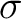electron correlation, goes in the opposite direction, allowing shorter bond distances for double bonds. For the C-C double bonds, these contrasting behaviors compensate each other  resulting in quite an accurate value for the bond length at the-CASSCF level. On the contrary, the extreme sensitivity of the C=O bond length to the electron correlation effects, leads to a general underestimation of the C-O double bond lengths, especially when such a bond is part of a conjugated system. It is indeed the effectiveness of the CASPT2 method in recovering dynamical correlation which leads to a substantial improvement in predicting the C-O double bond length.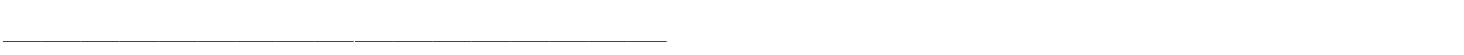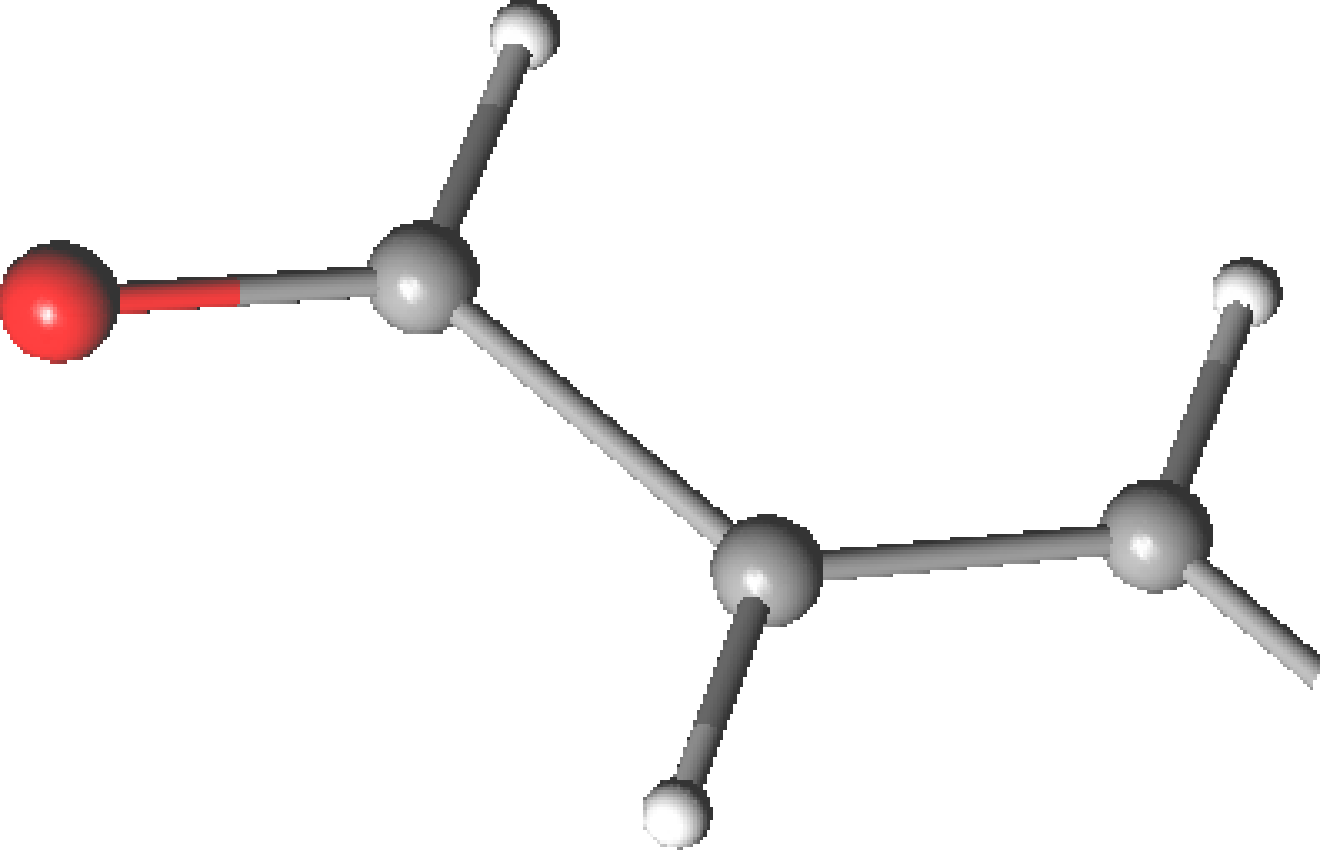Parametersa-CASSCF [04/4]-CASPT2 Expt.b s-cis s-trans s-cis s-trans C1=O 1.204 1.204 1.222 1.222 1.219 C1–C2 1.483 1.474 1.478 1.467 1.470 C2=C3 1.340 1.340 1.344 1.344 1.345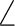C1C2C3 123.0 121.7 121.9 120.5 119.8C2C1O 124.4 123.5 124.5 124.2 - aBond distances in Å and angles in degrees. bMicrowave spectroscopy data from ref. . No difference between s-cis and s-trans isomers is reported

The use of numerical CASPT2 gradients can be extended to all the optimizations available in SLAPAF, for instance transition state searches. Use the following input for the water molecule to locate the linear transition state:

&GATEWAY;  Title=  Water,  STO-3G  Basis  set
Coord
3

H1  -0.761622  0.000000  -0.594478
H2  0.761622  0.000000  -0.594478
O  0.000000  0.000000  0.074915
Basis  set=  STO-3G
Group=  NoSym

>>>  EXPORT  MOLCAS_MAXITER=500
>>  DO  WHILE

&SEWARD

>>>  IF  (  ITER  =  1  )  <<<
&SCF;  Title=  water,  STO-3g  Basis  set
Occupied=  5
>>>  ENDIF  <<<

&RASSCF
Nactel=  2  0  0
Inactive=  4
Ras2  =  2

&CASPT2

&SLAPAF;  TS
>>>  ENDDO  <<<

After seventeen macro-iterations the linear water is reached:

*****************************************************************************************************************
* Energy Statistics for Geometry Optimization *
*****************************************************************************************************************
Iter Energy Change Norm Max Element Max Element Final Energy Update Update Index
1 -75.00567587 0.00000000 0.001456-0.001088 nrc003 -0.003312 nrc001 -75.00567822 RSIRFO None 1
2 -75.00567441 0.00000145 0.001471-0.001540 nrc003 -0.004162 nrc001 -75.00567851 RSIRFO MSP 1
3 -75.00566473 0.00000968 0.003484-0.002239 nrc003 0.008242 nrc003 -75.00567937 RSIRFO MSP 1
4 -75.00562159 0.00004314 0.006951-0.004476 nrc003 0.016392 nrc003 -75.00568012 RSIRFO MSP 1
5 -75.00544799 0.00017360 0.013935-0.008809 nrc003 0.033088 nrc003 -75.00568171 RSIRFO MSP 1
6 -75.00475385 0.00069414 0.027709-0.017269 nrc003 0.066565 nrc003 -75.00568219 RSIRFO MSP 1
7 -75.00201367 0.00274018 0.054556-0.032950 nrc003 0.084348* nrc003 -75.00430943 RSIRFO MSP 1
8 -74.99610698 0.00590669 0.086280-0.050499 nrc003 0.082995* nrc003 -74.99970484 RSIRFO MSP 1
9 -74.98774224 0.00836474 0.114866-0.065050 nrc003 0.080504* nrc003 -74.99249408 RSIRFO MSP 1
10 -74.97723219 0.01051005 0.139772 0.076893 nrc002 0.107680* nrc003 -74.98534124 RSIRFO MSP 1
11 -74.95944303 0.01778916 0.167230 0.096382 nrc002 -0.163238* nrc002 -74.97296260 RSIRFO MSP 1
12 -74.93101977 0.02842325 0.182451-0.114057 nrc002 0.185389* nrc002 -74.94544042 RSIRFO MSP 1
13 -74.90386636 0.02715341 0.157427-0.107779 nrc002 0.201775* nrc002 -74.91601550 RSIRFO MSP 1
14 -74.88449763 0.01936873 0.089073-0.064203 nrc002 0.240231 nrc002 -74.89232405 RSIRFO MSP 1
15 -74.87884197 0.00565566 0.032598-0.019326 nrc002 0.050486 nrc002 -74.87962885 RSIRFO MSP 1
16 -74.87855520 0.00028677 0.004934-0.004879 nrc003 -0.006591 nrc003 -74.87857157 RSIRFO MSP 1
17 -74.87857628 -0.00002108 0.000172-0.000120 nrc003 0.000262 nrc002 -74.87857630 RSIRFO MSP 1

+----------------------------------+----------------------------------+
+ Cartesian Displacements + Gradient in internals +
+ Value Threshold Converged? + Value Threshold Converged? +
+-----+----------------------------------+----------------------------------+
+ RMS + 0.1458E-03 0.1200E-02 Yes + 0.9925E-04 0.3000E-03 Yes +
+-----+----------------------------------+----------------------------------+
+ Max + 0.1552E-03 0.1800E-02 Yes + 0.1196E-03 0.4500E-03 Yes +
+-----+----------------------------------+----------------------------------+

Geometry is converged in 17 iterations to a Transition State Structure

*****************************************************************************************************************
*****************************************************************************************************************

We note that the optimization goes through three stages. The first one is while the structure still is very much ground-state-like. This is followed by the second stage in which the H-O-H angle is drastically changed at each iteration (iterations 7-13). The "*" at "Step Max" entry indicate that these steps were reduced because the steps were larger than allowed. Changing the default max step length from 0.3 to 0.6 (using keyword MaxStep) reduces the number of macro iterations by 2 iterations.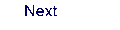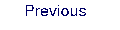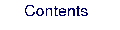Next: 10.3 Computing a reaction path. Up: 10. Examples Previous: 10.1 Computing high symmetry molecules.